#### How to Calculate Confidence Interval in Excel?

Excel is a powerful tool for various types of mathematical, engineering and statistical calculations and analyzes. It is up to you to take full advantage of the possibilities offered by Excel.

When preparing a statistical analysis, you usually do not work with data for the entire population. In most cases, you analyze the data calculated for the sample. So in the next step you need to calculate the confidence intervals.

In this article, I’ll show you how to do a confidence level analysis and explain how to use the confidence.norm function in Excel.

## What are Confidence Intervals?

The confidence interval for a given statistical measure tells us “how much we can trust a given value”. The confidence interval shows us that the real value is within the specified range with the assumed probability. The confidence interval is closely related to the theory of estimation in statistics.

Take, for example, the study of the mean for a given sample. We calculate the average value for the feature. In this way, by examining the sample mean, we try to determine the value of this feature for the entire population.

In order to estimate the value of a population feature, we establish confidence intervals for the value sought. Based on the sample examination, we can define the boundary ranges. These ranges include the value of the measure with the assumed probability.

Most often, 95% is used as the confidence interval, but another value, such as 99% or 80%, can also be used.

### Example of confidence intervals

Perhaps it is still not clear to you what this is all about. So let’s take an example. A high school teacher wanted to study the growth of students in the high school where he worked (population).

The school, however, was very large, so he decided to measure the students of only one class (samples). On this basis, using statistical knowledge, he determined the height of the students of the entire school.

By measuring the students across the class, he calculated that the average height of the students was 5 feet 5 inches.

This does not mean that this is the average height of all students in the school. Using statistical calculations, he determined the intervals (confidence intervals) and showed that the growth of students with a 95% probability. The true average height of all students throughout the school ranged from 5 feet 2 inches to 5 feet 8 inches.

## How the CONFIDENCE.NORM function works?

The Confidence.norm function will allow us to calculate both confidence level and confidence intervals.

### Syntax of Confidence.norrm function

=CONFIDENCE.NORM(alpha,standard_dev,size)

• alpha is the significance level that we use to define the confidence level. Alpha is usually 0.05. 95% is the most commonly used confidence level. The alpha is derived from the formula confidence level = 1 – alpha
• standard deviation is what you are able to know from a dedicated standard deviation tutorial
• size is a size of a sample so it is a number of observations you are having in your data set

## Calculating confidence intervals in Excel

### Calculations using Confidence.Norm function

You know a lot already about the topics. This is the time to try to calculate confidence levels in Excel.

We are curious on what is the confidence level and what are confidence intervals of salaries in our company. The calculation requires having data that looks like this.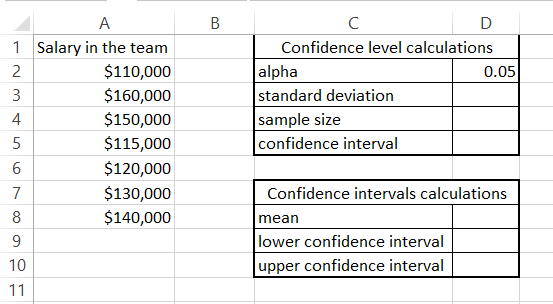First table contains what is needed for confidence level calculations:

• To calculate standard deviation for a sample just use =STDEV.S(A2:A8)
• Sample size is 7 but the formula to calculate it would be =COUNT(A2:A8)
• Confidence interval can be calculated by =CONFIDENCE.NORM(D2,D3,D4)

Having a confidence interval calculated you are able to set confidence intervals. To check confidence levels of a mean do calculate a mean first using the average function.

Based on mean value set confivence lower and upper levels with such a pattern:

• Lower confidence interval = mean – confidence interval
• Upper confidence interval = mean + confidence interval

In my example I used such a formulas:

• to calculate a mean =AVERAGE(A2:A8)
• Lower confidence interval =D8-D5
• Upper confidence interval =D8+D5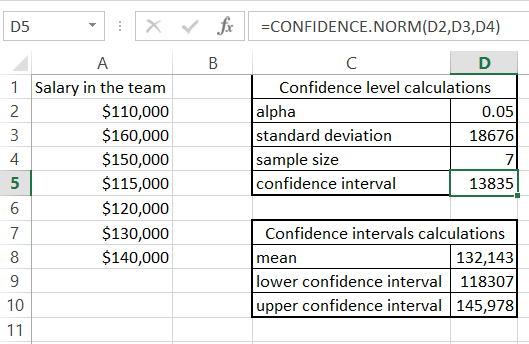Confidence intervals tells us than there is 95% probability that salary in the company is between 118307 and 145,978.

### Calculations using Analisys Toolpak add-in

It is possible to calculate confidence intervals using Analisys Toolpak add-in.

I have prepared data set of observation values. To calculate it go to the Ribbon > Data > Data Analysis. From the window which pops up pick Descriptive Statistics.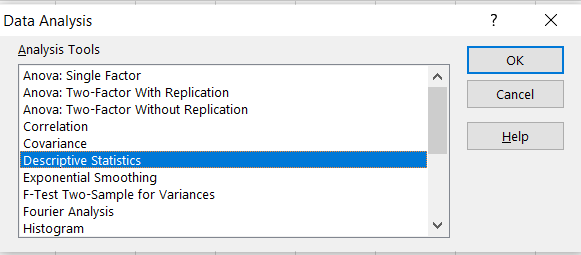As Input Range choose your data. It is \$B\$2:\$B\$11 for me.

An Output Range I would like to set \$E\$1. You can also choose New Worksheet Ply. I prefer to have it in existing sheet just next to my data set.

Two important things you need to tick are:

• Summary statistics to get summary output
• Confidence Level of Mean and choose the level which is 95% by default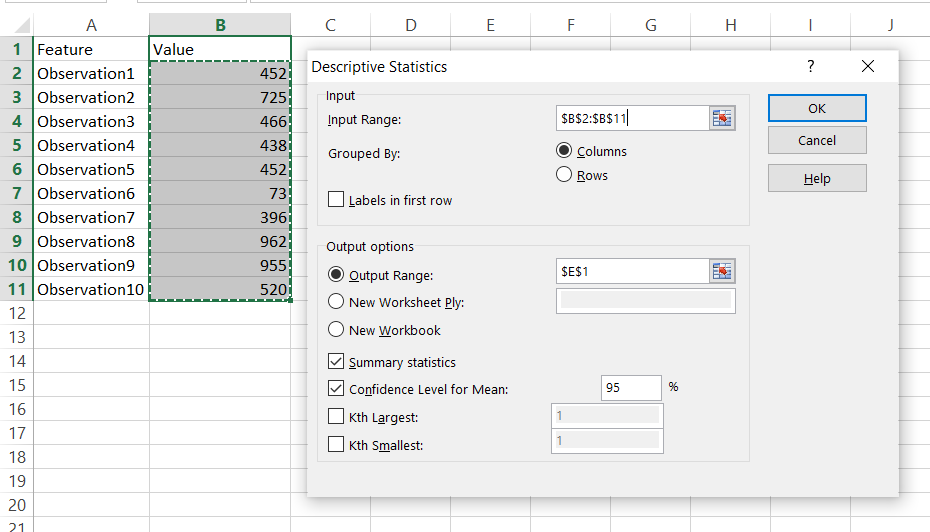This is how the summary looks like.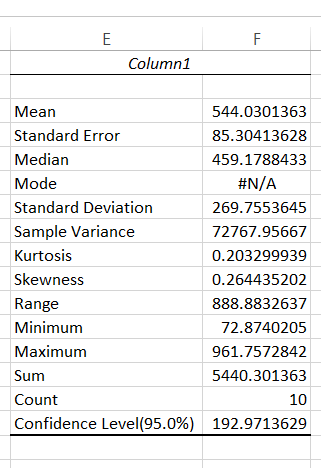Excel calculated:

The Analysis Toolpak returned all of these items, saving you a lot of effort. He has provided some information that may be useful to you. I recommend that you read the other stats articles on my site to become an expert.

## An alternative method using T.INV.2T function

Calculating a confidence interval in Excel can be done using the T.INV.2T function as well. The T.INV.2T function is used when you have a small sample size (less than 30) and the population standard deviation is unknown.

To calculate a confidence interval using the T.INV.2T function, follow these steps:

1. Enter your data into a column in Excel.
2. Calculate the sample mean by using the AVERAGE function.
3. Calculate the sample standard deviation by using the STDEV.S function.
4. Determine the sample size.
5. Determine the level of confidence you want to use (usually 95%).
6. Use the T.INV.2T function to calculate the margin of error. The formula is =T.INV.2T(alpha/2, df)*s/sqrt(n), where alpha is 1 minus the confidence level, df is the degrees of freedom (n-1), s is the sample standard deviation, and n is the sample size.
7. Calculate the upper and lower bounds of the confidence interval. The formula is =xbar +/- margin of error, where xbar is the sample mean.